www.batmath.it

### Exercises on determinants and linear systems

1. Find the determinants: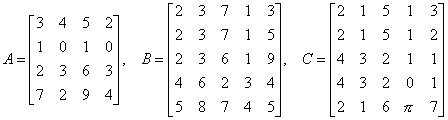.
2. If A and B are matrices of the same order and det(A)=2 and det(A)=3, find det(A2B-1).
3. Calculate the inverse: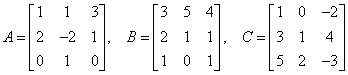.
4. Use Cramer's rule to solve the system: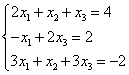.
5. Given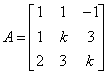, find the values of k for which det(A)=0. Solve the system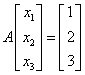.
6. Using Rouchè-Capelli's theorem solve the system: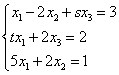, where s and t are real numbers.
7. Find the values of t for which the matrix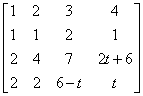is invertible.
8. Find the values of t for which the matrix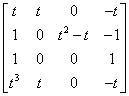is invertible.
first published on march 15 2002 - last updated on september 01 2003